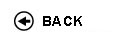Politecnico di Torino
Academic Year 2009/10
13AXOFL
Physics I
1st degree and Bachelor-level of the Bologna process in Environmental Engineering - Torino
 Teacher Status SSD Les Ex Lab Tut Years teaching Barbero GiovanniPO FIS/01 56 28 28 0 10
 SSD CFU Activities Area context FIS/01 7.5 A - Di base Fisica e chimica
 Objectives of the course This is a basic course that aims to teach basics of classical mechanics and thermodynamics and to enable students to solve simple problems. This course requires the knowledge acquired in the course of Mathematical analysis I. Beside lectures and practicals there are labs, that show students how to manage physical data. Expected skills Knowledge of mechanical and thermodynamic laws. Problem solving skills. Prerequisites Basic of differential and integral calsulus. Syllabus - Cartesian coordinates, polar, cylindrical, spherical. Motion of a point. Position, speed, acceleration. Uniform motion. Circular motion. Angular speed. Relative motion. - Inertial reference frames. Galilean principle of Relativity. Dynamic of a point. Mass and force. Newton's laws. Gravitational, Coulombian, elastic force. Acceleration on terrestrial surface. - Formal study of motion: 2nd Newton's law as differential equation system. Projectile motion. Harmonic motion. Simple pendulus. Moment of a force. Linear and angular moment. Kinetic and potential energy. Mechanical energy and its conservation. Etc. - Material point. Static and dynamic friction. Motion of a material point on a rectilinear or circular track, with or without friction. Simple pendulus. Equilibrium stability and its motion. - Mass geometry. Mass distribution. Huygens-Steiner's theorem. Etc. - Inner and outer forces. Cardinal equations of dynamics. Linear and angular moment of a mass system. Rigid systems. Axial moment. Roto-translation. Etc. Coriolis' acceleration. Relative dynamic. Coriolis' force. - Work. Power. Conservative and dissipative forces; potential energy. Kinetic energy of a rigid system. Etc. - Collisions. Impulsive forces. Elastic and inelastic forces. - Properties of a fluid. Incompressibility. Pressure. Ideal fluids. Equation of continuity. Bernouilli's theorem. Stevino's theorem. Archimede's principle. Viscosity. Etc. - Thermometric. Calorimetry. Transformations of a thermodynamic system. Reversibility and irreversibility. Perfect gases; inner and outer energy of perfect gases. Adiabatic, isotherm, isobar and isochor transformations. 1st and 2nd principle of thermodynamics. Carnot cycle. Carnot's and Clausius' theorems. Entropy. Etc. Laboratories and/or exercises - Practicals: 2 groups working on themes carried out during lectures. - Lab: 1 group: physical quantities. Physical laws. Dimensional analysis. Intrinsic uncertainty and errors. Errors. Histograms. Probability. Etc. 3 groups: simple pendulus, momentum of inertia, torsion pendulus. Check availability at the library Programma provvisorio per l'A.A.2005/06© Politecnico di Torino
Corso Duca degli Abruzzi, 24 - 10129 Torino, ITALY